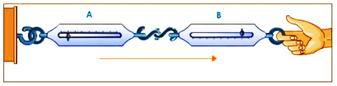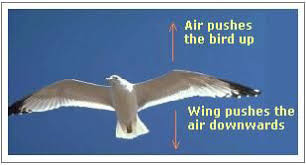# Newton's third law of motion explains the two forces namely action" and "reaction" coming into action when the two bodies are in contact with each other. These two forces:(a) Always act on the same body.(b)Always act on the different bodies in opposite directions.(c)Have the same magnitude and direction.(d)Acts on either body at normal to each other."

(b) Always act on the different bodies in opposite directions.

Explanation

Newtons' Third Law of motion states that "For every action, there is always an equal and opposite reaction"

We conclude the following points from Newton's Third Law of Motion:

1. In every interaction, forces always occur in pairs, means action and reaction always act on different objects.

2. The magnitude of these two forces are always equal.

3. The direction of the force on the first object A is opposite to the direction of the force on the second object B.

For example- If a bird exerts a force in a downward direction by pushing the air by its wings then it is the action force by the bird on air, whereas in return the air pushes the bird in the upward direction (opposite direction) as a reaction force which is the same amount of forces that were exerted by the bird in the downwards direction.